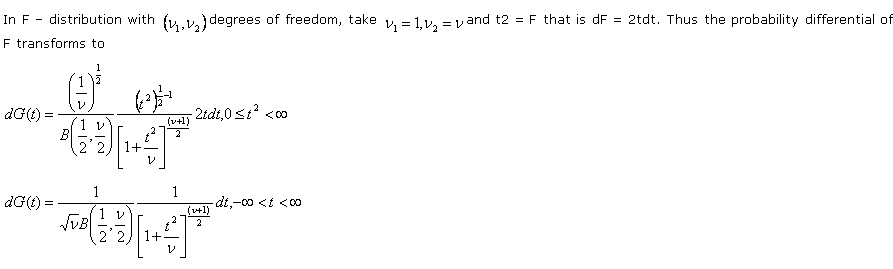# Statistics Assignment Help With Relation Between T And F Distributions

###The factor 2 disappearing since the total probability in the range (-∞, ∞) is unity. This is the probability function of student’s t – distribution with v degrees of freedom. Hence we have the following relation between t and F distributions.

If a statistic t follows student’s t distribution with n degrees of freedom then t2 follows Snedecor’s F – distribution with (1. N) degrees of freedom. Symbolically,

If t ~t(n) then t2 ~ F( 1, n)